Subtracting Decimals - Definition with Examples

The Complete K-5 Math Learning Program Built for Your Child

• 30 Million Kids

Loved by kids and parent worldwide

• 50,000 Schools

Trusted by teachers across schools

• Comprehensive Curriculum

Aligned to Common Core

Subtracting Decimals

Decimals numbers are the numbers that have a decimal point in them. It has two parts, the first is the number of wholes (left of the decimal point) and the second is fractional part (right of the decimal point).

For instance,
Let us consider that a bottle of milk costs \$4.80 (Decimal Number)
Here, the number of wholes = 4 (left of the decimal point)
Fractional part = 80 (right of the decimal point)Real-Life Applications:

• If the cost of 1 kg of an item is \$ 6.50 and we pay the shopkeeper \$ 7, then to work out the change, we will take away \$ 6.50 from \$ 7.
• To find the difference in the temperature of two different places.
• Scientists also use decimal numbers to obtain precise and accurate results in their experiments.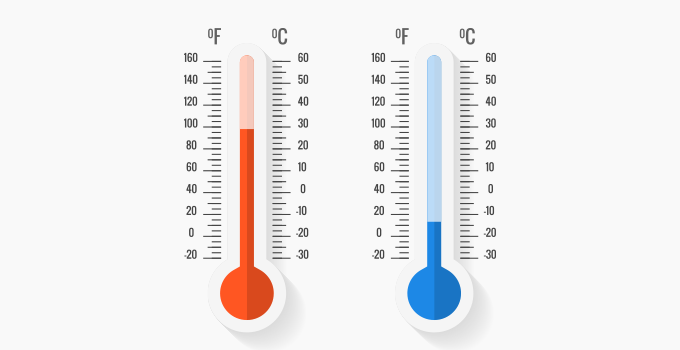Steps to subtract Decimal Number:

(i) Look at the decimal numbers we want to subtract.

(ii) Make sure both the decimal numbers have an equal number of digits after the decimal point. If not, we add extra 0 (Place-Value Holder) towards the end of the number, to make the digits after the decimal point equal.

(iii) Write both the numbers in the column subtraction format by lining up the decimal points of both the numbers, one above the other.

(iv) Subtract the decimals numbers just like we subtract whole numbers using the column subtraction. Make sure we line up the decimal digit in our answer appropriately.

(v) The number obtained is our answer.

Case-1: Two decimal numbers having an equal number of digits after the decimal point.

Example 1: Work out: 25.83 – 16.25

Solution:

 Step Number Observation Working Out Step (i) 25.83-16.25 Step (ii) Both of the numbers have equal number of digits after the decimal point. 25.83-16.25 Step (iii) Writing the calculation as column subtraction, line up the decimal points.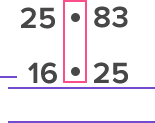Step (iv) Subtract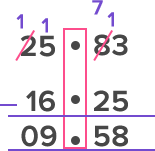Step (v) Answer 9.58

Hence, 25.83 – 16.25 = 9.58

Case-2: Two decimal numbers having different number of digits after the decimal point.

Example 2: The temperature of Cincinnati at 08:00 pm on Wednesday, April 17th was 32.78° C. By 04:00 am, the temperature dropped by 8.5° C. What was the temperature of Cincinnati at 04:00 am?

Solution:

 Step Number Observation Working Out Step (i) 32.78 – 8.5 Step (ii) Both of the numbers don’t have equal number of digits after the decimal point. So, we make them equal by adding a 0 after 8.5. 32.78 – 8.50 Step (iii) Write the calculation as column subtraction, and line up the decimal points.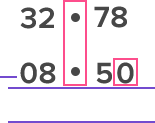Step (iv) Subtract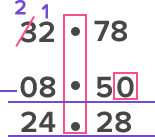Step (v) Answer 24.28

Hence, the temperature of Cincinnati at 04:00 am was 24.68º C

Case-3: When one of the numbers is decimal, and the other is a whole.

Example 3: Floki bought a ticket to Disneyland for \$46.85. If he paid \$50, how much change would he get back?

Solution:

 Step Number Observation Working Out Step (i) 50-46.85 Step (ii) Both of the numbers don’t have equal number of digits after the decimal point. So, we make them equal by adding a decimal point and two 0s after 50. 50.00-46.85 Step (iii) Write the calculation as column subtraction and line up the decimal points.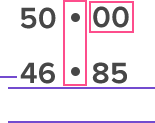Step (iv) Subtract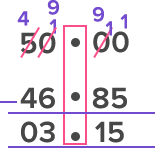Step (v) Answer 3.15

Hence, Floki gets a change of \$3.15.

 Fun Facts- The Chinese were the first people to use and develop fractions or decimal numbers.

Won Numerous Awards & Honors